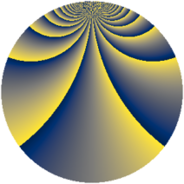# Properties

 Label 896.1.bmLevel $896$ Weight $1$ Character orbit 896.bm Rep. character $\chi_{896}(13,\cdot)$ Character field $\Q(\zeta_{32})$ Dimension $16$ Newform subspaces $1$ Sturm bound $128$ Trace bound $0$

# Related objects

## Defining parameters

 Level: $$N$$ $$=$$ $$896 = 2^{7} \cdot 7$$ Weight: $$k$$ $$=$$ $$1$$ Character orbit: $$[\chi]$$ $$=$$ 896.bm (of order $$32$$ and degree $$16$$) Character conductor: $$\operatorname{cond}(\chi)$$ $$=$$ $$896$$ Character field: $$\Q(\zeta_{32})$$ Newform subspaces: $$1$$ Sturm bound: $$128$$ Trace bound: $$0$$

## Dimensions

The following table gives the dimensions of various subspaces of $$M_{1}(896, [\chi])$$.

Total New Old
Modular forms 48 48 0
Cusp forms 16 16 0
Eisenstein series 32 32 0

The following table gives the dimensions of subspaces with specified projective image type.

$$D_n$$ $$A_4$$ $$S_4$$ $$A_5$$
Dimension 16 0 0 0

## Trace form

 $$16q + O(q^{10})$$ $$16q - 16q^{56} - 16q^{74} + O(q^{100})$$

## Decomposition of $$S_{1}^{\mathrm{new}}(896, [\chi])$$ into newform subspaces

Label Dim. $$A$$ Field Image CM RM Traces $q$-expansion
$$a_2$$ $$a_3$$ $$a_5$$ $$a_7$$
896.1.bm.a $$16$$ $$0.447$$ $$\Q(\zeta_{32})$$ $$D_{32}$$ $$\Q(\sqrt{-7})$$ None $$0$$ $$0$$ $$0$$ $$0$$ $$q-\zeta_{32}^{13}q^{2}-\zeta_{32}^{10}q^{4}-\zeta_{32}^{9}q^{7}+\cdots$$